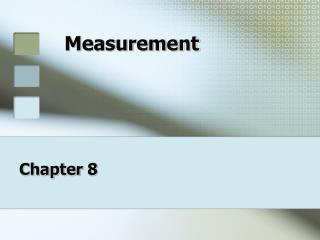DownloadDownload PresentationMeasurement

# Measurement

Download Presentation## Measurement

- - - - - - - - - - - - - - - - - - - - - - - - - - - E N D - - - - - - - - - - - - - - - - - - - - - - - - - - -
##### Presentation Transcript

1. Measurement Chapter 8

2. Why Measure? • Measurement is the practice of comparing the qualities of an object to a standard. • To describe objects using measurement, we have created systems of standards of comparison.

3. Early Measurements • Early Egyptians, Romans, and Greeks used the human body to start an early system of measurement. • Foot – originally based on the size of a human foot. • Cubit – Length of an Arm from the elbow to the tip of the middle finger (page 158). • Palm – Width of 4 Fingers • Digit – Width of a Single Finger.

4. Current Measurement Systems • Today, each major physical quality is compared to a standard measurement that has been set by government standards. • The two measurement standards in use in the world are: • U.S. Customary or English (feet, inches) • International System of Units (Metric)

5. U.S. (English) System • The U.S. customary system of measurement is based on the system that developed in England from approximately the 1100s to the 1500s. • The system is confusing because of inconsistencies in computing fractions and multiples of different base measurements. • For example, one inch is 1/12 of a foot, where as one quart is ¼ of a gallon.

6. U.S. (English) Measurement Some elements of ancient systems still remain in the words we use. • Size: • Inch – means thumb in some languages. • There are 12 inches in a foot • Mile – 5,280 feet. Comes from the length of one thousand paces of a Roman legion. • Volume: • Quart – 4 quarts in a gallon • Pint – 2 Pints in a quart

7. The Metric System • In the 1790s a group of French scientists assembled to create a measurement system that was more logical and exact. • The metric system is also simpler because it uses a prefix to show us how the base unit is being changed. For example the unit for distance is the meter. • The metric system uses the same prefixes for all base units. • The metric system is considered to be a more precise measurement system and is primarily used in medicine and other industry where precise measurement is needed.

8. Metric System Measurements • Everything is based on 10. • Size: • 10 millimeters in a centimeter • 10 centimeters in a decimeter • 10 decimeters in a meter • Volume: • 1,000 milliliters in a liter. • Weight: • 1,000 grams in a kilogram.

9. The second is the same for time measurement in both systems.

10. More About Measurement • Several distance measurements may be used when describing a part or product. • The U.S. customary system ISNOT built on the decimal system. • Precise measurements ARENOT usually needed when building a home. • Machinist’s rules are designed for finer measurements than bench rules. • A sliding T-bevel DOESNOT allow for a direct reading when measuring an angle. • The measurement tools an operator manipulates and reads ARENOT called indirect-reading measurement tools. • Mass is the amount of matter present in a material. • The gravitational pull exerted on an object is weight.

11. What can we measure? • Size and Space • Mass • Temperature • Time • Volume • Speed • Distance • Dimension

12. Size and Space • When we measure the size and shape of an object, we may encounter three different but related measurements: length or distance, area, and volume.

13. Measuring Length or Distance • Length is the separation between two points. • Length and distance are generally given in a single measurement from Point A to Point B. A • Many parts or products are described as having thickness, width, and height B Length: A & B Width Thickness

14. Measuring Area • Determining the area of something involves measuring both length and width and then multiplying the two to determine how much surface the object covers. L x W = Area Length Width

15. Measuring Volume • The amount of space that an object occupies or encloses • Volume is calculated by multiplying the length, width, and depth of an object. L x W x D = Volume • The measurement is expressed in cubic units. Width Depth Length

16. Types of Measurements • Standard Measurement: Measurement that is close but not exact. • Length of a piece of wood. • Building a House • Floor Covering • Precision Measurement: Measurements accurate to 1/1,000th of an inch (and smaller) • Machine Parts • Aircraft Components

17. Measurement Tools • Ruler – most common measurement tool – linear dimensions. • Protractor – measures angles. • Caliper – linear and diameter • Micrometer – used to measure linear dimensions. • Sliding T-bevel Square – DOES NOT allow for direct angle measurements. • Laser – indirect reading (processed by computer). • Optical Comparator - indirect reading (processed by computer).

18. Why Measure? • The foundation of a quality control system is measurement and analysis. Measurement is not used to measure just the output of a technology system, but all steps.